## Example Questions

2 Next →

### Example Question #21 : Plane Geometry

Suppose the side length of a pentagon is. What is the area of the pentagon?

Possible Answers: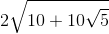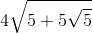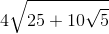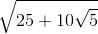Correct answer:Explanation:

Write the formula for the area of a pentagon.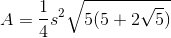Substitute the side length and simplify the radical.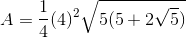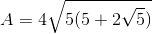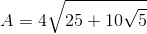### Example Question #1 : How To Find The Perimeter Of A Pentagon

One side of a regular pentagon is. Find the perimeter of the pentagon.

Possible Answers: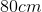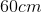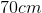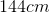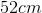Correct answer:Explanation:

In a regular pentagon, all of the sides are the same length.

The perimeter can then be found by summing allsides or multiplyingby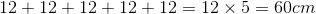### Example Question #2 : How To Find The Perimeter Of A Pentagon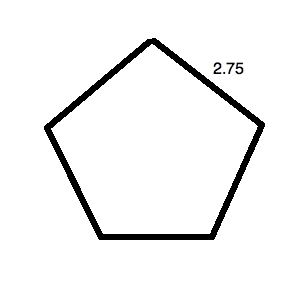What is the perimeter of the regular pentagon pictured above?

Possible Answers:

The answer cannot be determined.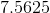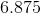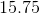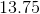Correct answer:Explanation:

The key word in this question is "regular." Since the pentagon is regular, all of its sides are equal. This means that it, in fact, looks like: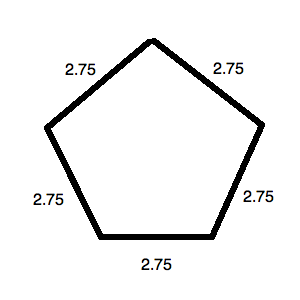The perimeter for this is simply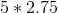or.

### Example Question #3 : How To Find The Perimeter Of A Pentagon

A regular pentagon has a side length of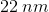. Find its perimeter.

Possible Answers: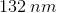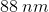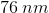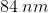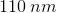Correct answer:Explanation:

Because we're told the pentagon is a regular polygon, this means that all of its sides are the same length. That is, the side lengths are congruent.

In order to solve for the perimeter, which is the sum of all sides, we can use a multiplication shortcut of: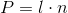, whereis perimeter,is length of the sides, andis the number of sides of the polygon.

Using the information given in the problem, the values can be substituted in.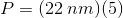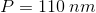Note that this math is the same as addingfive times: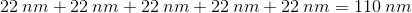### Example Question #1 : How To Find An Angle In A Pentagon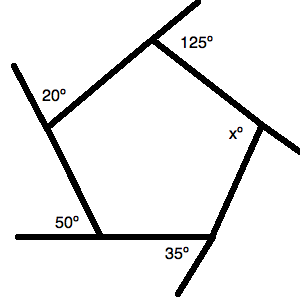The figure above is a pentagon.  All of the angles listed (except the interior one) are exterior angles to the pentagon's interior angles.

What is the value ofin the figure above?

Possible Answers: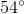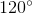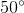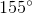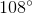Correct answer:Explanation:

There are two key things for a question like this. The first is to know that a polygon has a total degree measure of: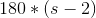, whereis the number of sides.

Therefore, a hexagon like this one has: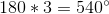Next, you should remember that all of the exterior angles listed are supplementary to their correlative interior angles. This lets you draw the following figure: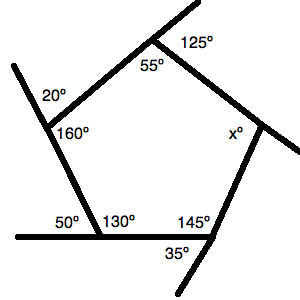Now, you just have to manage your algebra well. You must sum up all of the interior angles and set them equal to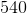. Thus, you can write: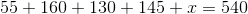Solve for: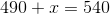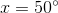### Example Question #1 : How To Find An Angle In A Pentagon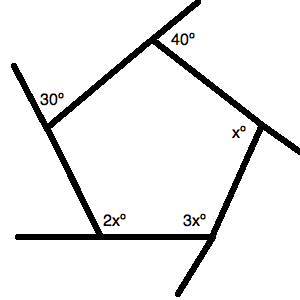The figure above is a pentagon.  All of the angles listed (except the interior one) are exterior angles to the pentagon's interior angles.

What is the value of the largest unknown angle in the figure above?

Possible Answers: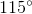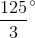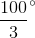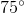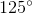Correct answer:Explanation:

There are two key things for a question like this. The first is to know that a polygon has a total degree measure of:, whereis the number of sides.

Therefore, a hexagon like this one has:.

Next, you should remember that all of the exterior angles listed are supplementary to their correlative interior angles. This lets you draw the following figure: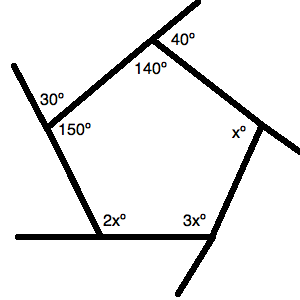Now, you just have to manage your algebra well. You must sum up all of the interior angles and set them equal to. Thus, you can write: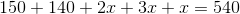Solve for: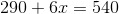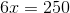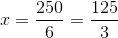Now, you have to find the largest unknown angle, which is: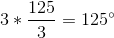### Example Question #3 : How To Find An Angle In A Pentagon

What is the sum of three angles in a pentagon?

Possible Answers: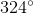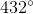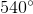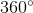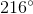Correct answer:Explanation:

The sum of all angles is determined by the following formula for a polygon: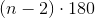In a pentagon, there are 5 sides, or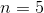.  Substitute and find the total possible angle in a pentagon.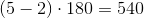There are 5 interior angles in a pentagon.  Divide the total possible angle by 5 to determine the value of one interior angle.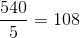Each interior angle of a pentagon is 108 degrees.

The sum of three angles in a pentagon is: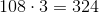2 Next →

### All ACT Math Resources